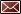Get Email Updates

Related Resources

Publications

# Interpolation of Proof from Table 1 of the Gauging Manual

## Correction Factors (Thermometers and Hydrometers)

Correction factors are numbers that convert the observed value into a corrected value. Correction values are determined by a third party vendor and displayed as a graph for both the thermometer and hydrometer. The correction factor may be zero but it must always be applied to the observed values recorded.

Sample Calibration Graph for a Hydrometer

Interpolation:

Interpolation is the process by which a value is calculated from within two known values. Specifically, Table 1 of the gauging manual is used to ascertain the proof at 60°F from the corrected hydrometer and thermometer readings and are displayed in whole number increments of 1 degree proof and 1°F. Because most readings, after correction factors are applied will be fractional, the proof and temperature have to be interpolated from Table 1.

Example:

The hydrometer reads 80.35 degrees proof at a temperature of 20.1 degrees Celsius. Your hydrometer has a correction factor of –0.03 and your thermometer has a correction factor of
+0.1.

 1 To apply the correction factor for proof you must subtract 0.03 from 80.35, the corrected proof will then be 80.32 degrees proof. To apply the correction factor for temperature you must add 0.1 to 20.1, the corrected temperature is 20.2 degrees Celsius. Convert 20.2 degrees Celsius to Fahrenheit, which is 68.36°F. 2 Take corrected values for proof and temperature and find the known values above and below from Table 1. For 80.32 degrees proof we need to find the values at 68°F: 80 proof at 68°F = 76.4°proof 81 proof at 68°F = 77.4°proof There is a 1.0 proof difference for the hydrometer. For 68.36°F we need to find the proof values at 80 proof: 80 proof at 68°F = 76.4°proof 80 proof at 69°F = 76.0°proof There is a –0.4 proof difference for temperature. “Minus” 0.4 because the proof goes down as the temperature increases. 3 Interpolate by multiplying the fractional parts of the hydrometer and thermometer readings by the differences determined above: Hydrometer: 0.32° x 1.0° = 0.32° Temperature: 0.36° x –0.4° = –0.144° 4 Use interpolated values to calculate the final proof: Final proof at 60°F = 76.4 + 0.32 + (–0.144) = 76.576. Round to the nearest tenth proof = 76.6°CONTACT US
Distilled Spirits Contacts
TTB desires to maintain a high level of communication with industry members, trade associations, State governments, and other Federal agencies.
For information regarding alcohol fuel, nonbeverage products, industrial alcohol, specially denatured alcohol, and tax free fuel, please view the information on the Other Alcohol pages.

Spirits Permits, Distilled Spirits Plant (DSP) or Alcohol Fuel Plant (AFP)
If you have questions regarding permits, applications, bonds, tax payments, etc., you may contact the National Revenue Center.

• Online Form: Submit an Inquiry
• Phone: 877-882-3277 (Toll Free)
• Mail: TTB National Revenue Center
550 Main Street, Room 8002
Cincinnati OH 45202